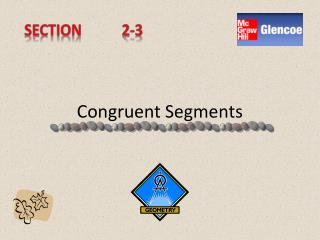DownloadDownload PresentationCongruent Segments

# Congruent Segments

Télécharger la présentation## Congruent Segments

- - - - - - - - - - - - - - - - - - - - - - - - - - - E N D - - - - - - - - - - - - - - - - - - - - - - - - - - -
##### Presentation Transcript

1. Section 2-3 Congruent Segments

2. In Geometry, two segments with the same length are called congruent segments. *Two segments are congruent if and only if they have the same length.*

3. Reading Geometry Read EG = FH as segment EG is congruent to segment FH. ~

4. Since congruence is related to the equality of segment measure, there are properties of congruence that are similar to the corresponding properties of equality. These statements are called theorems. Theorems are statements that can be justified by using logical reasoning.

5. There is a unique point on every segment called the midpoint. On the number line below, M is the midpoint of ST. What do you notice about SM and ST ?? S M T 1 2 3 4 5 6 7 8 -2 -1 0

6. Definition of Midpoint Words: A point M is the midpoint of a segment ST if and only if M is between S and T and SM=MT. Model: S M T Symbols: SM = MT

7. The midpoint of a segment separates the segment into two segments of equal length. So, by the definition of congruent segments, the two segments are equal in measure.

8. To bisect something means to separate it into two congruent parts. The midpoint of a segment bisects the segment because it separates the segment into two congruent segments. A point, line, ray, segment, or plane can also bisect a segment. Point F bisects EG. FD bisects EG. FA bisects EG. AC bisects EG. Plane ABC bisects EG. D C E F G A B

9. Hands-On Geometry Construction Materials: -straightedge -compass

10. Step 1 • Use a straightedge to draw the segment you wish to bisect. Name it XZ. X Z

11. Step 2 • Place the compass at point X. Use any compass setting greater than one half of XZ.Draw an arc above and below XZ. X Z

12. Step 3 • Using the same compass setting, place the compass at point Z. Draw an arc above and below XZ. These arcs should intersect the ones previously drawn. X Z

13. Step 4 • Use a straightedge to align the two intersections. Draw a segment that intersects XZ. Label the point of intersection Y. X Z Y